# Consecutive

#### Trigun1127

##### Member
Trying to get a Trend line to Plot a line across Highs (Bearish) and Lows (bullish) when each bar as a higher low for 5+ consecutive bars(bullish) or a lower High for 5+ consecutive bars (bearish). I would like that Line to Plot and update with every consecutive bar as soon as bar 5 closes. When the Low of a bar is breached(Bullish) it not longer updates until it finds the same sequence. The same for Lower Highs in a bear trend.

•Santhosh
Solution
@Trigun1127 might have a bug or two, didn't check too much.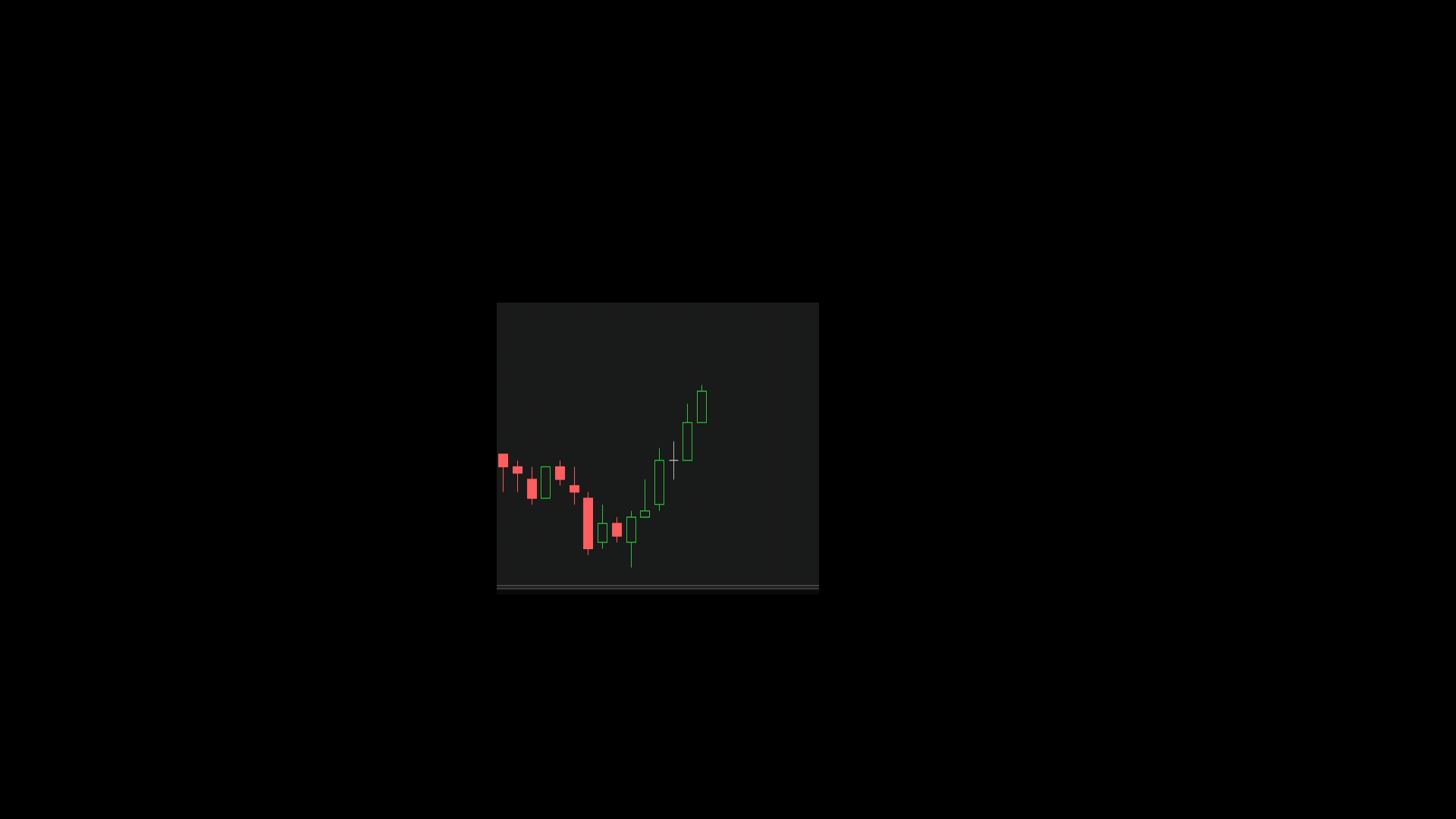Ruby:
``````input Number_Of_Consecutive_Lows = 5;
input Number_Of_Consecutive_Highs = 5;
input Reset_Time = 0930;

def Start ;
if GetAggregationPeriod() < AggregationPeriod.DAY {
Start = SecondsTillTime(Reset_Time) == 0;
} else {
Start = Double.NaN;}

def Bar = if !IsNaN(close) and BarNumber()>0 then BarNumber() else Bar;
########################################################################
#Do Not Remove Line of Code in this box.                               #
def FinalBar = if IsNaN(close[-1000]) then Bar[-1000] else FinalBar;#
########################################################################

def HigherLows;
if !IsNaN(Start) {
HigherLows...``````
Trying to get a Trend line to Plot a line across Highs (Bearish) and Lows (bullish) when each bar as a higher low for 5+ consecutive bars(bullish) or a lower High for 5+ consecutive bars (bearish). I would like that Line to Plot and update with every consecutive bar as soon as bar 5 closes. When the Low of a bar is breached(Bullish) it not longer updates until it finds the same sequence. The same for Lower Highs in a bear trend.

This should get you started.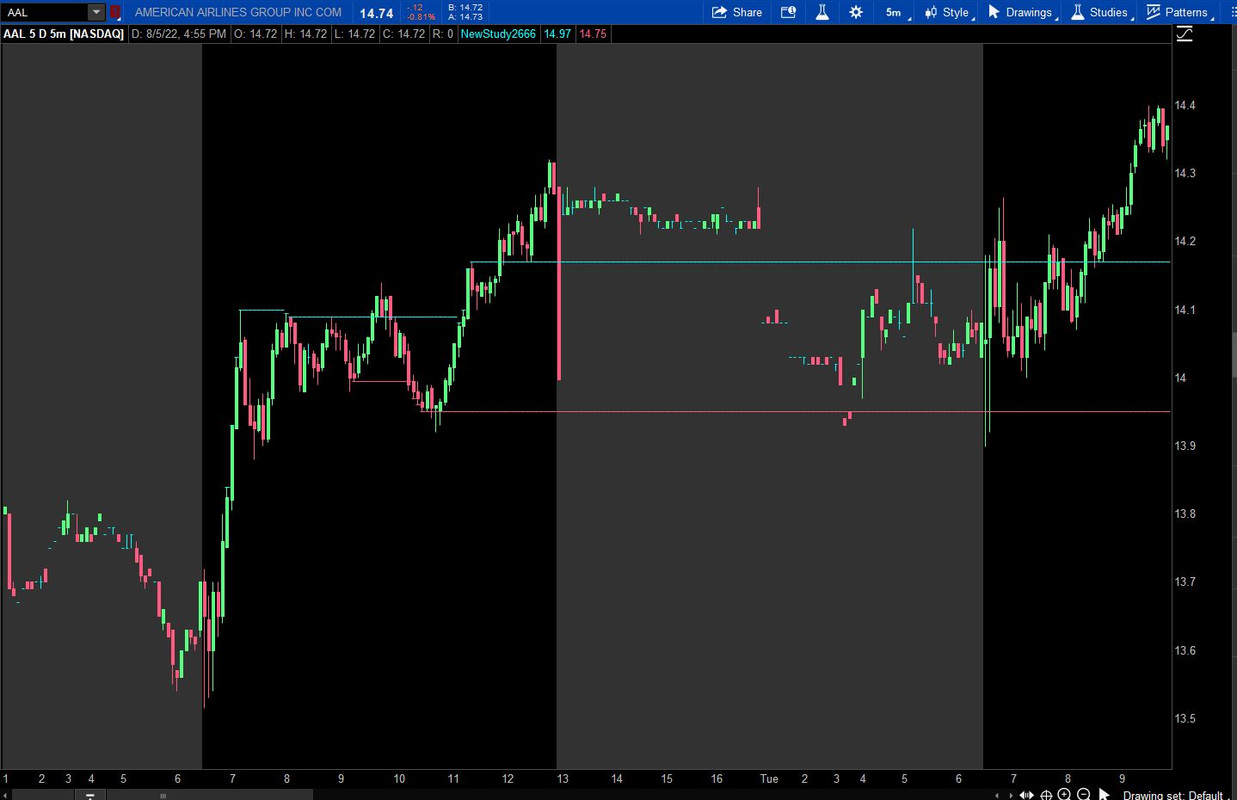Ruby:
``````def hlow   = if Sum(low > low, 5) >= 5 then high else hlow;
plot xhigh = hlow;
xhigh.SetPaintingStrategy(PaintingStrategy.HORIZONTAL);

def lhigh   = if Sum(high < high, 5) >= 5 then low else lhigh;
plot xlow   = lhigh;
xlow.SetPaintingStrategy(PaintingStrategy.HORIZONTAL);``````

I tried playing with it (don't know how to code). Was looking at an auto trend line script but I don't know the logic to plot those points. Here you can see what the script is doing and what it should look like. White Line is me and Blue line is script.

What was the original code used for that you posted? If you could help me out on this anymore I would really appreciate it.

@Trigun1127 might have a bug or two, didn't check too much.Ruby:
``````input Number_Of_Consecutive_Lows = 5;
input Number_Of_Consecutive_Highs = 5;
input Reset_Time = 0930;

def Start ;
if GetAggregationPeriod() < AggregationPeriod.DAY {
Start = SecondsTillTime(Reset_Time) == 0;
} else {
Start = Double.NaN;}

def Bar = if !IsNaN(close) and BarNumber()>0 then BarNumber() else Bar;
########################################################################
#Do Not Remove Line of Code in this box.                               #
def FinalBar = if IsNaN(close[-1000]) then Bar[-1000] else FinalBar;#
########################################################################

def HigherLows;
if !IsNaN(Start) {
HigherLows = if IsNaN(close) or SecondsTillTime(Reset_Time) == 0 then 0 else if low >= low then HigherLows+1 else 0;
}else {
HigherLows = if IsNaN(close) then 0 else if low >= low then HigherLows+1 else 0;}
def TargetHigherLows = if !IsNaN(Start) then if SecondsTillTime(Reset_Time)[-1] == 0 then 0 else fold z = 0 to Bar while GetValue(low,-(z+1)) >= GetValue(low,-z) do GetValue(HigherLows,-z-1)+1 else fold w = 0 to Bar while GetValue(low,-(w+1)) >= GetValue(low,-w) do GetValue(HigherLows,-w-1)+1;
def ConsecutiveHigherLowsEndBN = fold a = 0 to Bar while GetValue(low,-(a+1)) >= GetValue(low,-a) do GetValue(Bar,-a-1);
def ConsecutiveHigherLowsStartBN = if HigherLows == 0 then Bar else 0;
def ConsecutiveHigherLowsEndLow = fold c = 0 to Bar while GetValue(low,-(c+1)) >= GetValue(low,-c) do GetValue(low,-c-1);
def ConsecutiveHigherLowsStartLow = if HigherLows == 0 then low else 0;
def ConsecutiveHigherLowsSlope = if HigherLows == 0 then (ConsecutiveHigherLowsEndLow - ConsecutiveHigherLowsStartLow)/(ConsecutiveHigherLowsEndBN - ConsecutiveHigherLowsStartBN) else ConsecutiveHigherLowsSlope;
def ConsecutiveHigherLowsLine = if HigherLows == 0 then low else if low < low then Double.NaN else ConsecutiveHigherLowsLine + ConsecutiveHigherLowsSlope;

def LowerHighs;
if !IsNaN(Start) {
LowerHighs = if IsNaN(close) or SecondsTillTime(Reset_Time) == 0 then 0 else if high <= high then LowerHighs+1 else 0;
}else {
LowerHighs = if IsNaN(close) then 0 else if high <= high then LowerHighs+1 else 0;}

def TargetLowerHighs = if !IsNaN(Start) then if SecondsTillTime(Reset_Time)[-1] == 0 then 0 else fold y = 0 to Bar while GetValue(high,-(y+1)) <= GetValue(high,-y) do GetValue(LowerHighs,-y-1)+1 else fold x = 0 to Bar while GetValue(high,-(x+1)) <= GetValue(high,-x) do GetValue(LowerHighs,-x-1)+1;
def ConsecutiveLowerHighsEndBN = fold b = 0 to Bar while GetValue(high,-(b+1)) <= GetValue(high,-b) do GetValue(Bar,-b-1);
def ConsecutiveLowerHighsStartBN = if LowerHighs == 0 then Bar else 0;
def ConsecutiveLowerHighsEndHigh = fold d = 0 to Bar while GetValue(high,-(d+1)) <= GetValue(high,-d) do GetValue(high,-d-1);
def ConsecutiveLowerHighsStartHigh = if LowerHighs == 0 then high else 0;
def ConsecutiveLowerHighsSlope = if LowerHighs == 0 then (ConsecutiveLowerHighsEndHigh - ConsecutiveLowerHighsStartHigh)/(ConsecutiveLowerHighsEndBN - ConsecutiveLowerHighsStartBN) else ConsecutiveLowerHighsSlope;
def ConsecutiveLowerHighsLine = if LowerHighs == 0 then high else if high > high then Double.NaN else ConsecutiveLowerHighsLine + ConsecutiveLowerHighsSlope;

plot ConsecutiveHigherLowsPlot = if (TargetHigherLows >= Number_Of_Consecutive_Lows) or (HigherLows >= Number_Of_Consecutive_Lows) or (TargetHigherLows >= Number_Of_Consecutive_Lows and low >= low) then ConsecutiveHigherLowsLine else Double.NaN;
ConsecutiveHigherLowsPlot.SetStyle(Curve.MEDIUM_DASH);

plot ConsecutiveLowerHighsPlot = if (TargetLowerHighs >= Number_Of_Consecutive_Highs) or (LowerHighs >= Number_Of_Consecutive_Highs) or (TargetLowerHighs >= Number_Of_Consecutive_Highs and high <= high) then ConsecutiveLowerHighsLine else Double.NaN;
ConsecutiveLowerHighsPlot.SetStyle(Curve.MEDIUM_DASH);``````

Last edited:
••Trigun1127, Santhosh, SleepyZ and 1 other person
This indicator is working amazing for the most part. I've spotted 1 issues and just 1 minor rule change.
1. Today when plotting the line it took Yesterdays low and added it to the plot. I have extended hours turned off
2. If you could change the rule so that the low being equal to the prior bar is acceptable. I've attached 2 images of where it doesn't plot because the prior bar was equal either in High or low.

Another thing that happened was there was a line drawn here today but it just disappeared for some reason

It actually seems on more review that the line on average only plots once a day and doesnt plot again but on rare occasions.

It actually seems on more review that the line on average only plots once a day and doesnt plot again but on rare occasions.
-Your initial condition of 5 consecutive bars with higher lows or lower highs is why. The Higher the time frame, the less times it occurs. Lower time frames or lower the number of consecutive bars initially required will yield more plots. (Adding the 'or equal to' condition will yield more plots as well).

1. Today when plotting the line it took Yesterdays low and added it to the plot. I have extended hours turned off
2. If you could change the rule so that the low being equal to the prior bar is acceptable. I've attached 2 images of where it doesn't plot because the prior bar was equal either in High or low.
-Updated Code to include input for time of day to reset counters.
-Updated Code to include equal highs or lows. (Note: This may cause some separate lines to combine into a 'ziqzag' as two adjacent bars can be the end of one plot and the beginning of another.) I may have a work around for this, will have to experiment a little.

Another thing that happened was there was a line drawn here today but it just disappeared for some reason

Is it sad I recognized the chart for /ES in your images? Didn't know the time frame, but I was certain it was /ES

-I can't recreate this, the line plotted and stayed there for me.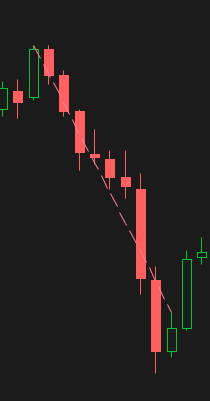Last edited:
•Trigun1127
Haha yes your right! except its /MES.
1. What you said about higher time frames I completely understand. Below you'll see some dates and times with examples where it should have plotted but didn't for some reason.
2. Actually so far I don't actually mind the zigzags I haven't come across any yet that have been bothersome to me but actually give some interesting results.
3. That picture you posted on my comment of the plot disappearing, your code made it reappear and that goes for many other plots that weren't showing as well.

5Min Time Frame
8-2-22 10:50 a.m.
8-3-22 2:00 p.m.
8-5-22 11:10 A.M.

Updated code again, Fixed those and a few more.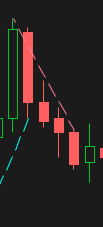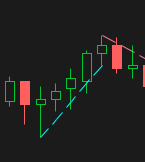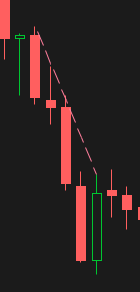#### Attachments

•Trigun1127
interesting. This indicator, does it re-paint?

So far brother its working perfectly fantastic job. Ill keep you updated If I see anything more. I really wish I could donate to you all for the effort you and the other coders put in for free.

Updated code again, Fixed those and a few more.Hey Svanoy the indicator has been working fantastically. Can I get a slightly modified code on the side so it can also display on a Higher time frame charts like Daily, Weekly? I think the the start time of 9:30 stops it from working.

#### Attachments

•RichG
@Trigun1127 updated code above to disregard the start time if the aggregation period is daily or above.

•Trigun1127
@Trigun1127 updated code above to disregard the start time if the aggregation period is daily or above.
can we do the same thing with closes above and MA?
so instead of higher lows its consecutive closes above an MA?

@Tiredoflosing

Ruby:
``````input Number_Of_Consecutive_Lows = 1;
input Number_Of_Consecutive_Highs = 1;

def MA = SimpleMovingAvg(close,50);

def Bar = if !IsNaN(close) and BarNumber()>0 then BarNumber() else Bar;
########################################################################
#Do Not Remove Line of Code in this box.                               #
def FinalBar = if IsNaN(close[-1000]) then Bar[-1000] else FinalBar;#
########################################################################
def Higher1 = close;
def Higher2 = MA;
def HigherLows = if IsNaN(close) then 0 else if Higher1 >= Higher2 then HigherLows+1 else 0;

def TargetHigherLows = fold z = 0 to Bar while GetValue(Higher1,-(z+1)) >= GetValue(Higher2,-z) do GetValue(HigherLows,-z-1)+1;
def ConsecutiveHigherLowsEndBN = fold a = 0 to Bar while GetValue(Higher1,-(a+1)) >= GetValue(Higher2,-a) do GetValue(Bar,-a-1);
def ConsecutiveHigherLowsStartBN = if HigherLows == 0 then Bar else 0;
def ConsecutiveHigherLowsEndLow = fold c = 0 to Bar while GetValue(Higher1,-(c+1)) >= GetValue(Higher2,-c) do GetValue(low,-c-1);
def ConsecutiveHigherLowsStartLow = if HigherLows == 0 then low else 0;

def ConsecutiveHigherLowsSlope = if HigherLows == 0 then (ConsecutiveHigherLowsEndLow - ConsecutiveHigherLowsStartLow)/(ConsecutiveHigherLowsEndBN - ConsecutiveHigherLowsStartBN) else ConsecutiveHigherLowsSlope;
def ConsecutiveHigherLowsLine = if HigherLows == 0 then low else if Higher1 < Higher2 then Double.NaN else ConsecutiveHigherLowsLine + ConsecutiveHigherLowsSlope;

def Lower1 = close;
def Lower2 = MA;
def LowerHighs = if IsNaN(close) then 0 else if Lower1 <= Lower2 then LowerHighs+1 else 0;

def TargetLowerHighs = fold y = 0 to Bar while GetValue(Lower1,-(y+1)) <= GetValue(Lower2,-y) do GetValue(LowerHighs,-y-1)+1;
def ConsecutiveLowerHighsEndBN = fold b = 0 to Bar while GetValue(Lower1,-(b+1)) <= GetValue(Lower2,-b) do GetValue(Bar,-b-1);
def ConsecutiveLowerHighsStartBN = if LowerHighs == 0 then Bar else 0;
def ConsecutiveLowerHighsEndHigh = fold d = 0 to Bar while GetValue(Lower1,-(d+1)) <= GetValue(Lower2,-d) do GetValue(high,-d-1);
def ConsecutiveLowerHighsStartHigh = if LowerHighs == 0 then high else 0;
def ConsecutiveLowerHighsSlope = if LowerHighs == 0 then (ConsecutiveLowerHighsEndHigh - ConsecutiveLowerHighsStartHigh)/(ConsecutiveLowerHighsEndBN - ConsecutiveLowerHighsStartBN) else ConsecutiveLowerHighsSlope;
def ConsecutiveLowerHighsLine = if LowerHighs == 0 then high else if Lower1 > Lower2 then Double.NaN else ConsecutiveLowerHighsLine + ConsecutiveLowerHighsSlope;

plot ConsecutiveHigherLowsPlot = if (TargetHigherLows >= Number_Of_Consecutive_Lows) or (HigherLows >= Number_Of_Consecutive_Lows) or (TargetHigherLows >= Number_Of_Consecutive_Lows and Higher1 >= Higher2) then ConsecutiveHigherLowsLine else Double.NaN;
ConsecutiveHigherLowsPlot.SetStyle(Curve.MEDIUM_DASH);

plot ConsecutiveLowerHighsPlot = if (TargetLowerHighs >= Number_Of_Consecutive_Highs) or (LowerHighs >= Number_Of_Consecutive_Highs) or (TargetLowerHighs >= Number_Of_Consecutive_Highs and Lower1 <= Lower2) then ConsecutiveLowerHighsLine else Double.NaN;
ConsecutiveLowerHighsPlot.SetStyle(Curve.MEDIUM_DASH);``````

Last edited:
@Tiredoflosing

Ruby:
``````input Number_Of_Consecutive_Lows = 1;
input Number_Of_Consecutive_Highs = 1;

def MA = SimpleMovingAvg(close,50);

def Bar = if !IsNaN(close) and BarNumber()>0 then BarNumber() else Bar;
########################################################################
#Do Not Remove Line of Code in this box.                               #
def FinalBar = if IsNaN(close[-1000]) then Bar[-1000] else FinalBar;#
########################################################################
def Higher1 = close;
def Higher2 = MA;
def HigherLows = if IsNaN(close) then 0 else if Higher1 >= Higher2 then HigherLows+1 else 0;

def TargetHigherLows = fold z = 0 to Bar while GetValue(Higher1,-(z+1)) >= GetValue(Higher2,-z) do GetValue(HigherLows,-z-1)+1;
def ConsecutiveHigherLowsEndBN = fold a = 0 to Bar while GetValue(Higher1,-(a+1)) >= GetValue(Higher2,-a) do GetValue(Bar,-a-1);
def ConsecutiveHigherLowsStartBN = if HigherLows == 0 then Bar else 0;
def ConsecutiveHigherLowsEndLow = fold c = 0 to Bar while GetValue(Higher1,-(c+1)) >= GetValue(Higher2,-c) do GetValue(low,-c-1);
def ConsecutiveHigherLowsStartLow = if HigherLows == 0 then low else 0;

def ConsecutiveHigherLowsSlope = if HigherLows == 0 then (ConsecutiveHigherLowsEndLow - ConsecutiveHigherLowsStartLow)/(ConsecutiveHigherLowsEndBN - ConsecutiveHigherLowsStartBN) else ConsecutiveHigherLowsSlope;
def ConsecutiveHigherLowsLine = if HigherLows == 0 then low else if Higher1 < Higher2 then Double.NaN else ConsecutiveHigherLowsLine + ConsecutiveHigherLowsSlope;

def Lower1 = close;
def Lower2 = MA;
def LowerHighs = if IsNaN(close) then 0 else if Lower1 <= Lower2 then LowerHighs+1 else 0;

def TargetLowerHighs = fold y = 0 to Bar while GetValue(Lower1,-(y+1)) <= GetValue(Lower2,-y) do GetValue(LowerHighs,-y-1)+1;
def ConsecutiveLowerHighsEndBN = fold b = 0 to Bar while GetValue(Lower1,-(b+1)) <= GetValue(Lower2,-b) do GetValue(Bar,-b-1);
def ConsecutiveLowerHighsStartBN = if LowerHighs == 0 then Bar else 0;
def ConsecutiveLowerHighsEndHigh = fold d = 0 to Bar while GetValue(Lower1,-(d+1)) <= GetValue(Lower2,-d) do GetValue(high,-d-1);
def ConsecutiveLowerHighsStartHigh = if LowerHighs == 0 then high else 0;
def ConsecutiveLowerHighsSlope = if LowerHighs == 0 then (ConsecutiveLowerHighsEndHigh - ConsecutiveLowerHighsStartHigh)/(ConsecutiveLowerHighsEndBN - ConsecutiveLowerHighsStartBN) else ConsecutiveLowerHighsSlope;
def ConsecutiveLowerHighsLine = if LowerHighs == 0 then high else if Lower1 > Lower2 then Double.NaN else ConsecutiveLowerHighsLine + ConsecutiveLowerHighsSlope;

plot ConsecutiveHigherLowsPlot = if (TargetHigherLows >= Number_Of_Consecutive_Lows) or (HigherLows >= Number_Of_Consecutive_Lows) or (TargetHigherLows >= Number_Of_Consecutive_Lows and Higher1 >= Higher2) then ConsecutiveHigherLowsLine else Double.NaN;
ConsecutiveHigherLowsPlot.SetStyle(Curve.MEDIUM_DASH);

plot ConsecutiveLowerHighsPlot = if (TargetLowerHighs >= Number_Of_Consecutive_Highs) or (LowerHighs >= Number_Of_Consecutive_Highs) or (TargetLowerHighs >= Number_Of_Consecutive_Highs and Lower1 <= Lower2) then ConsecutiveLowerHighsLine else Double.NaN;
ConsecutiveLowerHighsPlot.SetStyle(Curve.MEDIUM_DASH);``````
Thank you for this! do u know if this is repainting overall?
Also, shouldnt the line start after "n" closes above the sma?

@Tiredoflosing I've not looked at it much after coding it, not sure about repainting or not, would definitely recommend higher inputs than the default. The line is plotted by calculation of slope, so it plots from the points used to calculate it.

### Not the exact question you're looking for?

87k+ Posts
249 Online## The Market Trading Game Changer

Join 2,500+ subscribers inside the useThinkScript VIP Membership Club
• Exclusive indicators
• Proven strategies & setups
• Private Discord community
• Exclusive members-only content
• 1 full year of unlimited support

What is useThinkScript?

useThinkScript is the #1 community of stock market investors using indicators and other tools to power their trading strategies. Traders of all skill levels use our forums to learn about scripting and indicators, help each other, and discover new ways to gain an edge in the markets.

How do I get started?

We get it. Our forum can be intimidating, if not overwhelming. With thousands of topics, tens of thousands of posts, our community has created an incredibly deep knowledge base for stock traders. No one can ever exhaust every resource provided on our site.

If you are new, or just looking for guidance, here are some helpful links to get you started.

What are the benefits of VIP Membership?